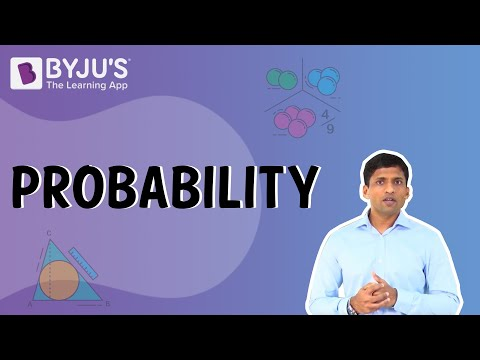# Probability Class 9 Notes - Chapter 15

## Introduction to Probability

### Probability

• Probability is the measure of the likelihood of an event to occur. Events can’t be predicted with certainty but can be expressed as to how likely it can occur using the idea of probability.
• Probability can range between 0 and 1, where 0 probability means the event to be an impossible one and probability of 1 indicates a certain event.To know more about Probability, visit here.

### Experiment

An experiment:

• is any procedure that can be infinitely repeated or any series of actions that have a well-defined set of possible outcomes.
• can either have only one or more than one possible outcomes.
• is also called the sample space.

### Trail

• A single event that is performed to determine the outcome is called a trial.
• All possible trials that constitute a well-defined set of possible outcomes are collectively called an experiment/sample space.

## Experimental Probability

### Experimental/Empirical Probability

The empirical probability of an event that may happen is given by:

Probability of event to happen P(E)=Number of favourable outcomes/Total number of outcomes

To know more about Experimental Probability, visit here.

### Coin Tossing Experiment

Consider a fair coin. There are only two possible outcomes that are either getting heads or tails.

Number of possible outcomes = 2
Number of outcomes to get head = 1
The probability of getting head =Number of outcomes to get head/Number of possible outcomes=1/2

### Rolling of Dice Experiment

When a fair dice is rolled, the number that comes up top is a number between one to six. Assuming we roll the dice once, to check the possibility of three coming up.

Number of possible outcomes = 6
Number of outcomes to get three = 1
The probability of getting three = Number of outcomes to get three/Number of possible outcomes=1/6

### Sum of Probabilities of Favorable and Unfavourable events

• When a trial is done for an expected outcome, there are chances when the expected outcome is achieved. Such a trial/event is called a favourable event.
• When a trial is done for an expected outcome, there are chances when the expected outcome is not achieved. Such a trial/event is called an unfavourable event.
• All favourable and unfavourable event outcomes come from the well-defined set of outcomes.
• Suppose an event of sample space S has n favourable outcomes. Then, there are S-n, unfavourable outcomes.
• The probability of favourable and unfavourable events happening depends upon the number of trials performed. However, the sum of both these probabilities is always equal to one.
Test your Knowledge on Probability Class 9